BeamLab’s Mode Solver is a versatile tool to design and analyze optical waveguides and photonic integrated circuits in your familiar MATLAB® environment. In its basic configuration, it calculates the eigenmodes and corresponding effective refractive indices of any waveguide’s two-dimensional refractive index distribution with high precision. In addition, it provides you with the possibility to calculate important waveguide parameters such as the bend loss, group delay, effective mode area, dispersion parameter, and many more as a function of the wavelength. It can also be used in conjunction with BeamLab’s BPM Solver to generate input field distributions based on waveguide eigenmodes.

Mode Solver is based on the Finite Difference Method which solves the eigenvalue matrix equations for the electric field derived from finite-difference approximations of the semi- or full-vectorial wave equation. All solutions with regard to field distributions are obtained on a rectangular (Cartesian) mesh as MATLAB® matrices and are thus well suited for efficient visualization and post-processing.

MATLAB® environment

• Intuitive user interface directly integrated in the MATLAB® environment
• Solve complex optical problems with just a few lines of code
• No hassle with learning a new proprietary language
• No need for third-party APIs for transferring your data to and from MATLAB®
• Platform-independent (Windows, macOS, Linux)• Highly optimized algorithms implemented natively in MATLAB®
• Full-vectorial and semi-vectorial mode solver algorithms based on the finite difference method
• 3D or 2D analysis
• Various types of boundary and symmetry conditions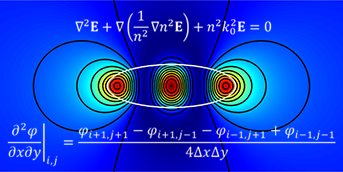Waveguide dispersion

• Simulation of many different dispersion characteristics as a function of the normalized frequency or wavelength
• Analysis of effective refractive index, normalized propagation constant, effective mode area, and mode field diameter
• Analysis of dispersion parameter, modal group delay, and differential group delay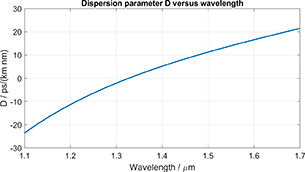Anisotropy

• Analysis of waveguide bends
• Analysis of anisotropic waveguides with different anisotropies in different waveguide parts (e.g., core, substrate and cladding)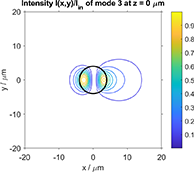Utmost simulation flexibility

• Large variety of easy-to-use waveguide functions
• High flexibility in post-processing and editing any output data and graphs
• Refractive index database for common optical materials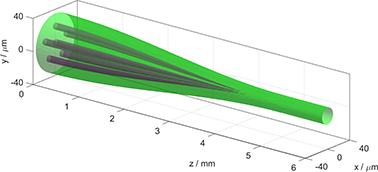Powerful graphics

• Harnessing the vast visualization options of the MATLAB® environment
• 1D, 2D, and 3D plots with any color and shading you can imagine
• Pseudocolor and contour plots
• Illustrative quiver plots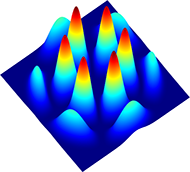Parameter sweeps and optimization problems

• Flexible MATLAB® programming language to implement any kind of parameter sweep
• Powerful MATLAB® optimization functions such as fminsearch to optimize your photonic devices
• Parameterize any variable for your sweeps and optimizations, e.g., waveguide geometry, refractive indices, wavelength, and many more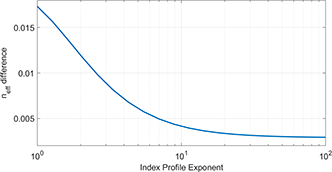Parallel computing

• Parallel computing using multicore processors (requires MATLAB® Parallel Computing Toolbox™).

Application Gallery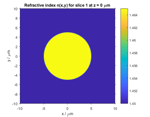Fiber Modes

Eigenmode calculation of an optical fiber
View demo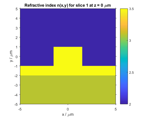Rib Waveguide Modes

Eigenmode calculation of a rib waveguide
View demo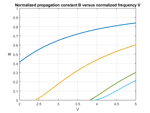Fiber Dispersion

Dispersion evaluation of an optical fiber
View demo